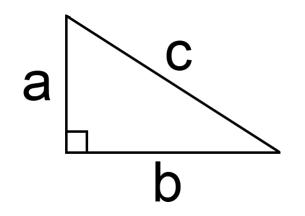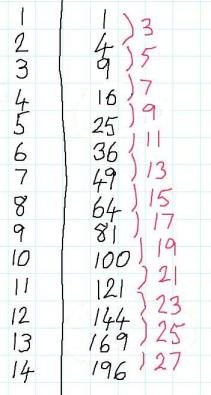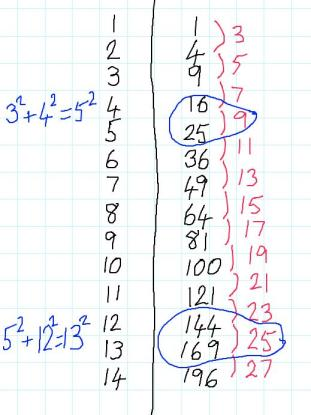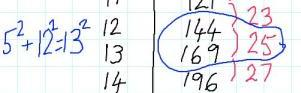#### You may also like### Odd Differences

The diagram illustrates the formula: 1 + 3 + 5 + ... + (2n - 1) = n² Use the diagram to show that any odd number is the difference of two squares.### Triangular Triples

Show that 8778, 10296 and 13530 are three triangular numbers and that they form a Pythagorean triple.# Generating Triples

### Why do this problem?

This problem connects different areas of mathematics. Students are required to apply their knowledge of Pythagoras' theorem and properties of numbers, while using their algebra skills to make generalisations and prove their conjectures.

### Possible approach

"Look at this right-angled triangle:""We know that the three sides have to satisfy the relationship $a^2 + b^2 = c^2$.
Can you find examples where $a, b$ and $c$ are whole numbers? Here is a table of square numbers you might find useful."Give students a few minutes to seek sets of 3 numbers. Then bring the class together again and collect the examples they found on the board. If ${3, 4, 5}$ and ${6, 8, 10}$ have both been suggested, it may be worth taking the time to discuss whether we should be surprised that doubling a, b and c still maintained the Pythagorean relationship.

For students who are wondering why the difference between consecutive square numbers is always odd, this representation might be useful.

Now introduce the name Pythagorean triple to describe a set of three whole numbers that satisfy $a^2 + b^2 = c^2$.

"To start with, let's focus on Pythagorean Triples where b and c differ by just 1. The annotations on this table might give you some ideas on how to find some more."Set students to work in pairs looking for more Pythagorean triples. "At the end of the lesson, I want you to be able to explain a method for generating more Pythagorean triples where $b$ and $c$ differ by just 1, and to be able to explain how you know your method will always work."

For some students, it may be appropriate to nudge them towards algebraic representations to justify their findings. This could start with writing the relationship as $a^2 + b^2 = (b+1)^2$
to explain why $a$ needs to be an odd number.

Then students could move on to the more sophisticated idea of writing $a^2$ as $(2n+1)^2$ and using their results to find expressions for $b$ and $c$ in terms of $n$.

If more lesson time is available, students can go on to use their insights from investigating the case where $b$ and $c$ differ by 1 to explore triples where they differ by more than 1. There are some suggestions for follow-up questions in the problem.

### Key questions

Look at the table:Where is the $5^2$?
Where is the $12^2$?
Where is the $13^2$?

Where might we find a similar set of related square numbers?

### Possible support

What's Possible? offers a rich exploration of properties of square numbers that could be a good starting point for working on this problem.

### Possible extension

Few and Far Between? challenges students to find triples where $a$ and $b$ differ by just 1.